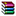# [用MATLAB写算法]之排序算法1)插入排序

filename: insertion_sort

%%%%%%%%%%%%%%%%%%%%%%%%%%%%%%%%%%%%

function sorted_array=insertion_sort(num_array)
% sorted_array=insertion_sort(num_array)  ascending
% algorithm complexity: theta(n^2)

for i=2:length(num_array)
for j=1:i-1
if num_array(i)<num_array(j)
tmp=num_array(i);
for k=i-1:-1:j
num_array(k+1)=num_array(k);
end
num_array(j)=tmp;
break
end
end
end

sorted_array=num_array;

%%%%%%%%%%%%%%%%%%%%%%%%%%%%%%%%%%%%%insertion_sort.m.zip

http://blog.sciencenet.cn/blog-71294-1039179.html

## 相关博文

GMT+8, 2021-4-14 21:13

Powered by ScienceNet.cn

Copyright © 2007- 中国科学报社